# Optimization of computational algorithms

(diff) ← Older revision | Latest revision (diff) | Newer revision → (diff)

The choice of an optimal computational algorithm in the solution of applied problems or in the elaboration of systems of standard programs. When solving a concrete problem, the optimal tactic can be not to optimize the solution method, but to opt for a standard program or to use the simplest method for which the composition of a program is reasonably straightforward.

The theoretical formulation of the question of optimization of computational algorithms is based on the following principles. When choosing a method for solving a problem, the researcher concentrates on certain properties, and his choice of the algorithm depends on these, while the algorithm will also be used to solve other problems possessing these properties. For this reason, in the theoretical study of algorithms one introduces a class of problemswhich possess specific properties. When choosing a solution method, the researcher has a setof solution methods at his disposal. When using a methodto solve a problem, the solution obtained will have a certain error. The quantity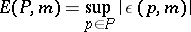is called the error of the methodin the class, while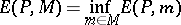is called the optimal estimate of the error infor the methods from. If a method exists such that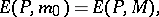then this method is said to be optimal. A scheme of studying the problem of optimization of computational algorithms, dating back to A.N. Kolmogorov , considers the set of problems of computing the integral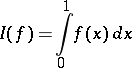given the condition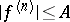, and whereis the set of all possible quadratures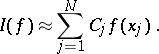Each quadrature is defined by the totality of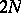numbersand. The problem of the minimum quantity of information (see , ) needed to recover a function from a given class with required accuracy can also be included in this scheme. A more complex formulation of the problem is examined in , in which the amount of work involved in realizing the algorithm in a specific sense is commensurate with the amount of memory used. Optimal algorithms exist for only an insignificant number of type of problems . However, for a large number of computational problems, methods have been created which are almost optimal in their asymptotic characteristics (see ).

Research into the characteristics of computational algorithms which are optimal in their class (see , ) comprises two parts: creating concrete solution methods with the best possible characteristics, and obtaining estimates from below of the characteristics of computational algorithms (see , ). In essence, the first part of the question is a basic problem of the theory of numerical methods and in most cases it is studied independently of the optimization problem. Obtaining estimates from below usually reduces to an estimation from below of the-entropy or of the width of the corresponding spaces; this is sometimes carried out independently, using the same techniques as are used in obtaining the estimates mentioned.

Computational algorithms may be divided into passive ones and active ones. A passive algorithm for solving a problem does not depend on the information obtained in solving the problem, whereas an active algorithm does. When computing an integral, the information used about the function is usually information on its values atpoints. For a passive algorithm, the integral is computed using the formula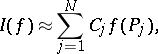where the weights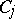andfrom the domainof definition ofare previously defined. Active algorithms for computing the integral are included in the following scheme: A point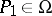and the functions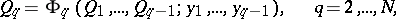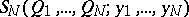are given, where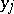,are numbers,. The following quantities are successively computed: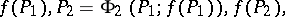and it is supposed that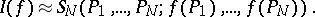In convex classes of integrands which are centrally symmetric with respect to the function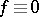, the optimal estimate in the class of passive algorithms coincides with the optimal estimate in the class of active algorithms (see , ).

In the practice of numerical integration, active algorithms of the type of integration algorithms with automatic choice of steps (see ) have shown their superiority over passive algorithms. This supports the generally held point of view that a formal scheme of optimization of computational algorithms does not often include specific real problems. When solving optimization problems (especially minimization problems), passive algorithms are hardly used (see , ). As an upper bound for the amount of work involved in calculating one value of a function from some classone implicitly takes the unit of amount of work of the algorithm. There are other possible approaches to estimating the optimality of the characteristics of an algorithm. For example, the amount of work involved in computing a functional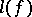from a set of functionalscan be taken as the unit of amount of work. In this case, a lower bound for the amount of work involved in realizing the algorithms is obtained using the theory of widths (see , ). The amount of work comprises not only the amount of work involved in obtaining information on initial data, but also the amount of work involved in processing the information. At the present time, it appears that one cannot cite examples of classes of real computational problems in which lower bounds for the amount of work involved in realizing the algorithms can be obtained which differ from the bounds on the information of the type under consideration. However, for a number of non-computational problems of this type, bounds are known (see Algorithm, computational complexity of an and Algorithm, complexity of description of an).

When research into problems of optimization of computational algorithms aims to solve problems on a computer, the problem has extra nuances related to the stability of the algorithm in relation to the error of computation, and to the restriction on the volume of the different forms of memory used (see Computational algorithm). The problem of optimizing computational algorithms has been treated above as a problem of optimization of computational algorithms in a class of problems. In practice, the problem of optimization of computational algorithms for a concrete problem is of vital interest (see , ). The formulation of one problem of optimization (see ) is as follows. A differential equation is integrated using the Runge–Kutta method with a variable step. An estimate is made of the principal term of the estimation error. This estimate is then optimized through a distribution of the points of integration (in general, for a given number of points). This approach to the problem of optimization has a crucial bearing on the development of the theory and on the practice of active algorithms of numerical integration.

How to Cite This Entry:
Optimization of computational algorithms. Encyclopedia of Mathematics. URL: http://encyclopediaofmath.org/index.php?title=Optimization_of_computational_algorithms&oldid=14278
This article was adapted from an original article by N.S. Bakhvalov (originator), which appeared in Encyclopedia of Mathematics - ISBN 1402006098. See original article